# 3 Digit Addition with Regrouping

3 Digit Addition with Regrouping – Question 1

Add the following: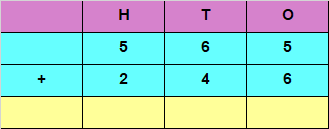Explanation

Adding the digits at ones place,
5 + 6 = 11
Since the number is more than 9, we carry over 1 to the tens place,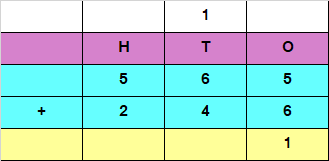Adding the digits at tens place, and the carryover of 1
6 + 4 + 1 = 11
Since the number is more than 9, we carry over 1 to the hundreds place,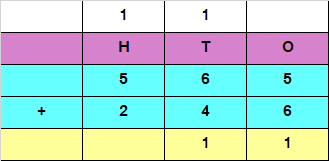Adding the digits at hundreds place, and the carryover of 1
5 + 2 + 1 = 8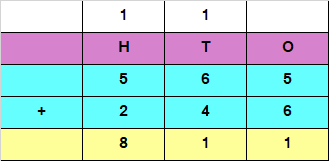Hence, the sum of the given numbers is 811

3 Digit Addition with Regrouping – Question 2

​Add the following: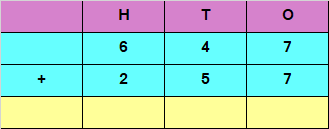Explanation

Adding the digits at ones place,
7 + 7 = 14
Since the number is more than 9, we carry over 1 to the tens place,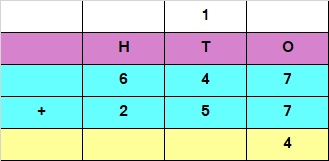Adding the digits at tens place and the carry of 1
1 + 4 + 5 = 10
Since the number is more than 9, we carry over 1 to the hundreds place,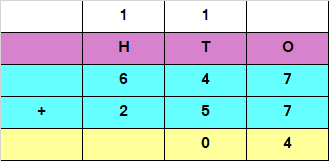Adding the digits at hundreds place and the carry of 1
6 + 2 + 1 = 9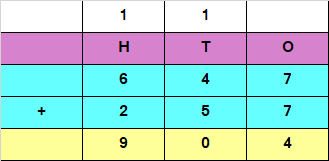Hence, the sum of the given numbers is 904